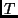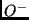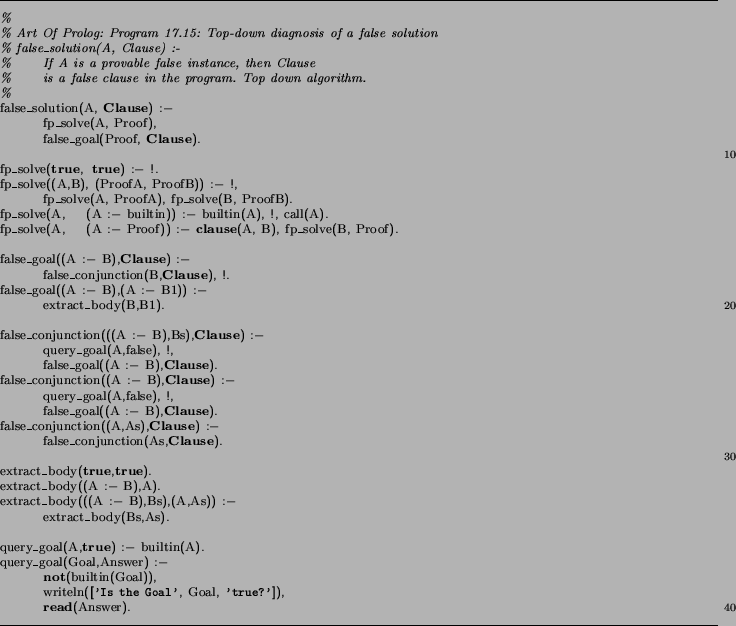Next: Refinement Up: COMP9417 Project Shapiro's Model Previous: Introduction

Subsections

The contradiction backtracing algorithm is the most interesting part of Shapiro's algorithmic error diagnosis framework [Sha83], as it has some philosophical implications for the refinement of scientific theories [Sha81]. This algorithm exploits the simple logical semantics of pure Prolog, and so an understanding of refutation theorem proving in general and the resolution calculus specifically is required (Section 1.2) to understand this section.

The following inputs are required:

• A theorythat proves a negative observation. We say thatis overly-general; it is unsound relative to the concept the oracle has in mind.

• An oracle capable of answering queries about the truth of ground atoms in the model.

The core of the algorithm is the following: as pure Prolog has only one mechanism for getting work done (a SLDNF-resolution step: unification of a body goal with the head of a clause), there are only a very few possible sources of bugs. In particular, ifcan prove, then it must be the case that there is at least one clause whose head is asserted false by the oracle, and whose body is deemed to be true in(there is a proof of this assertion in [Sha83, p38]).

Given this characteristic of the faulty clause, diagnosing incorrect solutions boils down to searchingfor one that has it. [Sha83, Section 3.2] presents several querying strategies with varying query complexity, which is defined to be the asymptotic number of times the oracle is consulted in terms of the size of the computation (proof) tree. In effect, this metric measures the data-hungriness of this diagnosis algorithm.

The search implemented by our applet is top down, shown in Figure 2 and described in [SS94, p337] like so:

...At each node it tries to find a false [child]. The algorithm recurses with any false [child] found. If there is no false [child], then the current node constitutes a counterexample [and indicates the faulty clause], as the goal at the node is false and all of its [children] are true.
It should be apparent that this algorithm queries the oracle a number of times that is a linear function of the depth of the proof tree.

Shapiro proves that his divide-and-query strategy (not presented here) is query-optimal, that is, it makes the most efficient use of the oracle for this task. The idea is an application of the classic divide-and-conquer paradigm, and bears a striking similarity to binary search: imagine the proof tree as a linear structure; query the node that lies nearest the middle, then recurse in the half where the bug must lie. This algorithm is logarithmic in the number of nodes in the proof tree.Once such a bug has been discovered, the Model Inference System retracts the clause that has been identified as the culprit of the missing solution, and marks it as being unsatisfactory. A later pass will refine such marked clauses into more specific ones (Section 3).

 Your browser does not support Java applets.

## Other Diagnosis Algorithms

While the backtracing algorithm can determine which clause is responsible for the generation of a false solution, logic programs can exhibit other unwanted behaviour:

• Finitely failing to prove something that it should be able to. Shapiro developed a missing solution algorithm that localises the cause of such bugs.

• Exhausting the stack during a proof attempt. There is a stack overflow algorithm that determines the cause of this mis-behaviour.

Shapiro has presented (some of) these algorithms in at least three places [Sha81,Sha83,SS94], with varying degrees of sophistication. These algorithms are useful for declarative debugging [Llo87a], independently of their usefulness in machine learning.Next: Refinement Up: COMP9417 Project Shapiro's Model Previous: Introduction
Peter Gammie
2002-03-01